IMO Shortlist 2014 problem N6

Kvaliteta:
Avg: 0,0
Težina:
Avg: 8,0

Let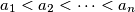$a_1 < a_2 < \cdots < a_n$ be pairwise coprime positive integers with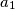$a_1$ being prime and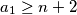$a_1 \geq n + 2$. On the segment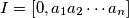$I = [0, a_1 a_2 \cdots a_n]$ of the real line, mark all integers that are divisible by at least one of the numbers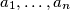$a_1, \ldots, a_n$. These points split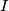$I$ into a number of smaller segments. Prove that the sum of the swquares of the length of these segments is divisible by$a_1$.

(Serbia)

Izvor: https://www.imo-official.org/problems/IMO2014SL.pdf